• Call Now

1800-102-2727•

# Velocity-Definition, Types, Practice Problems, FAQs

## What is Velocity?

The change in position vector or the displacement of a body divided by the time taken is called velocity. In the following diagram, the position vector of the body changes from point A to B. Displacement is defined as the shortest possible distance between two points.Motion between two points A and B

Imagine yourself cycling in a circular path of radius r as shown in figure.

Distance between points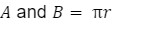Displacement between points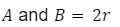It is a vector quantity. Velocity carries a SI unit of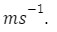Velocity of an object is said to be positive if the body moves in the positive direction, and negative if it moves in the negative direction.

## Velocity vs Speed

Though both of them have the same units, yet they are totally different. Speed is scalar, whereas velocity is a vector.

## Average Velocity

The total displacement covered by a body divided by the total time taken is called average velocity(vavg). In the following displacement time (s-t) graph, the average velocity in the time interval ab is equal to the slope of the graph between a and b.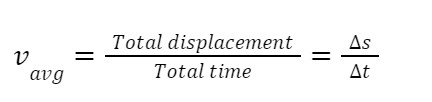s indicates the displacement undergone in time t.

## Instantaneous Velocity

The velocity of an object(v) at a particular instant of time is called “instantaneous velocity vinst ”. If ds indicates displacement in time dt, then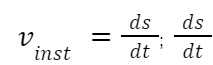indicates the derivative of displacement with respect to time at a given point, P in our case.

The graphical meaning of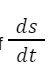at point P is the slope of the graph at point P i.e the slope of the tangent drawn on the s-t curve at point P. Direction of instantaneous velocity at an instant is the same as the direction of average velocity for a straight-line motion if the body does not reverse its direction of motion. Direction of instantaneous velocity at an instant may be opposite to the direction of average velocity for a straight line motion if the body reverses its direction of motion.## Uniform and Non-Uniform Motion

A motion is said to be uniform if the body covers equal distance in equal intervals of time. For eg. if a body covers 2 m of distance each second, the body is said to be in uniform motion. Whereas a motion is said to be non-uniform if the body covers unequal distances in equal intervals of time. A body in non-uniform motion is said to have acceleration.

## Relative Velocity

Motion is never absolute; it is always “relative”. It can only be defined wrt an observer. So, relative velocity is defined as the velocity of one body with respect to another.

The relative velocity of A wrt B can be written as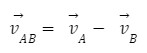## Practice Problems of Velocity

Question 1.  A car A moves along east with a velocity of 20 ms-1. Another car B moves west with a speed of 40 ms-1. The relative velocity of A wrt B has magnitude

(a)20 ms-1 (b)10 ms-1 (c)60 ms-1 (d) 25 ms-1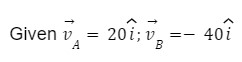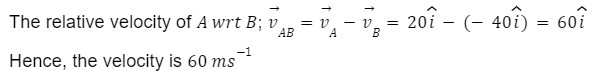Question 2.  A police van is moving on a highway with a speed of 30 kmhr-1 fires a bullet at a thief car which is moving away in the same direction with a speed of 190 kmhr-1. If the muzzle speed of the bullet is 150 ms-1, find the speed of the bullet with respect to the thief's car.

Answer: Muzzle velocity of the bullet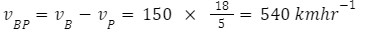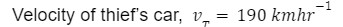Since bullet is fired from the police car, the velocity of bullet,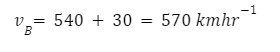Velocity of the bullet with respect to the thief,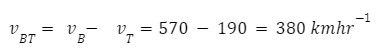Question 3. A particle moves along the x-axis. At a time t, the displacement is given by x=40+12t-t3. It’s x coordinate when it comes to rest?

(a) 24 m  (b) 40 m (c) 56 m (d) 16 m

When it comes to rest, velocity=0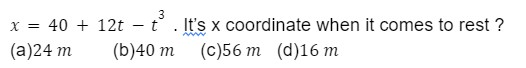Question 4.  A car travels with a velocity vu from X to Y. It returns from Y to X with a velocity vd. What is the average velocity of the car during this entire trip?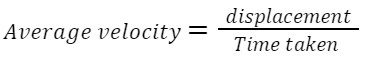Since the displacement for the entire trip is zero, average velocity is zero.=

## FAQs of Velocity

Question 1. What is velocity?

Answer: Velocity is defined as the change in displacement divided by the change in time.

Question 2. Is velocity a scalar or vector quantity?

Answer: Velocity is a vector quantity. It has direction.

Question 3.  What is the difference between velocity and speed?

Answer: Velocity is total displacement divided by the total time taken. Speed is the total distance divided by the total time taken. Speed is a scalar quantity whereas velocity is a vector quantity.

Question 4.  Is average velocity greater than average speed?

Answer: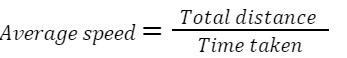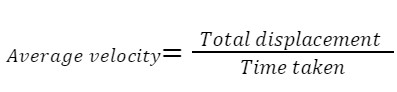Since distance is greater than or equal to the magnitude of displacement, average speed is greater than or equal to average velocity.

## NCERT Class 11 Physics Chapters

 Physical World Units and Measurements Motion in a Straight Line Motion in a Plane Laws of Motion Work Energy and Power Particles and Rotational Motion Gravitation Mechanical Properties of Solids Mechanical Properties in Liquids Thermal Properties of Matter Thermodynamics Kinetic Theory Oscillations WavesTalk to our expert
Resend OTP Timer =
By submitting up, I agree to receive all the Whatsapp communication on my registered number and Aakash terms and conditions and privacy policy